Prealgebra 2e

# 4.5Add and Subtract Fractions with Different Denominators

Prealgebra 2e4.5 Add and Subtract Fractions with Different Denominators

### Learning Objectives

By the end of this section, you will be able to:

• Find the least common denominator (LCD)
• Convert fractions to equivalent fractions with the LCD
• Add and subtract fractions with different denominators
• Identify and use fraction operations
• Use the order of operations to simplify complex fractions
• Evaluate variable expressions with fractions

### Be Prepared 4.12

Before you get started, take this readiness quiz.

Find two fractions equivalent to $56.56.$
If you missed this problem, review Example 4.14.

### Be Prepared 4.13

Simplify: $1+5·322+4.1+5·322+4.$
If you missed this problem, review Example 4.48.

### Find the Least Common Denominator

In the previous section, we explained how to add and subtract fractions with a common denominator. But how can we add and subtract fractions with unlike denominators?

Let’s think about coins again. Can you add one quarter and one dime? You could say there are two coins, but that’s not very useful. To find the total value of one quarter plus one dime, you change them to the same kind of unit—cents. One quarter equals $2525$ cents and one dime equals $1010$ cents, so the sum is $3535$ cents. See Figure 4.7.

Figure 4.7 Together, a quarter and a dime are worth $3535$ cents, or $3510035100$ of a dollar.

Similarly, when we add fractions with different denominators we have to convert them to equivalent fractions with a common denominator. With the coins, when we convert to cents, the denominator is $100.100.$ Since there are $100100$ cents in one dollar, $2525$ cents is $2510025100$ and $1010$ cents is $10100.10100.$ So we add $25100+1010025100+10100$ to get $35100,35100,$ which is $3535$ cents.

You have practiced adding and subtracting fractions with common denominators. Now let’s see what you need to do with fractions that have different denominators.

First, we will use fraction tiles to model finding the common denominator of $1212$ and $13.13.$

We’ll start with one $1212$ tile and $1313$ tile. We want to find a common fraction tile that we can use to match both $1212$ and $1313$ exactly.

If we try the $1414$ pieces, $22$ of them exactly match the $1212$ piece, but they do not exactly match the $1313$ piece.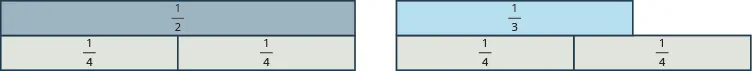If we try the $1515$ pieces, they do not exactly cover the $1212$ piece or the $1313$ piece.If we try the $1616$ pieces, we see that exactly $33$ of them cover the $1212$ piece, and exactly $22$ of them cover the $1313$ piece.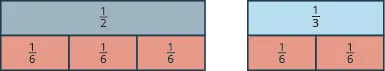If we were to try the $112112$ pieces, they would also work.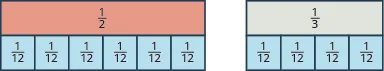Even smaller tiles, such as $124124$ and $148,148,$ would also exactly cover the $1212$ piece and the $1313$ piece.

The denominator of the largest piece that covers both fractions is the least common denominator (LCD) of the two fractions. So, the least common denominator of $1212$ and $1313$ is $6.6.$

Notice that all of the tiles that cover $1212$ and $1313$ have something in common: Their denominators are common multiples of $22$ and $3,3,$ the denominators of $1212$ and $13.13.$ The least common multiple (LCM) of the denominators is $6,6,$ and so we say that $66$ is the least common denominator (LCD) of the fractions $1212$ and $13.13.$

### Manipulative Mathematics

Doing the Manipulative Mathematics activity "Finding the Least Common Denominator" will help you develop a better understanding of the LCD.

### Least Common Denominator

The least common denominator (LCD) of two fractions is the least common multiple (LCM) of their denominators.

To find the LCD of two fractions, we will find the LCM of their denominators. We follow the procedure we used earlier to find the LCM of two numbers. We only use the denominators of the fractions, not the numerators, when finding the LCD.

### Example 4.63

Find the LCD for the fractions $712712$ and $518.518.$

### Try It 4.125

Find the least common denominator for the fractions: $712712$ and $1115.1115.$

### Try It 4.126

Find the least common denominator for the fractions: $13151315$ and $175.175.$

To find the LCD of two fractions, find the LCM of their denominators. Notice how the steps shown below are similar to the steps we took to find the LCM.

### How To

#### Find the least common denominator (LCD) of two fractions.

1. Step 1. Factor each denominator into its primes.
2. Step 2. List the primes, matching primes in columns when possible.
3. Step 3. Bring down the columns.
4. Step 4. Multiply the factors. The product is the LCM of the denominators.
5. Step 5. The LCM of the denominators is the LCD of the fractions.

### Example 4.64

Find the least common denominator for the fractions $815815$ and $1124.1124.$

### Try It 4.127

Find the least common denominator for the fractions: $13241324$ and $1732.1732.$

### Try It 4.128

Find the least common denominator for the fractions: $928928$ and $2132.2132.$

### Convert Fractions to Equivalent Fractions with the LCD

Earlier, we used fraction tiles to see that the LCD of $1414$ when $1616$ is $12.12.$ We saw that three $112112$ pieces exactly covered $1414$ and two $112112$ pieces exactly covered $16,16,$ so

$14=312 and 16=212.14=312 and 16=212.$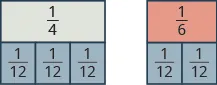We say that $1414$ and $312312$ are equivalent fractions and also that $1616$ and $212212$ are equivalent fractions.

We can use the Equivalent Fractions Property to algebraically change a fraction to an equivalent one. Remember, two fractions are equivalent if they have the same value. The Equivalent Fractions Property is repeated below for reference.

### Equivalent Fractions Property

If $a,b,ca,b,c$ are whole numbers where $b≠0,c≠0,b≠0,c≠0,$ then

$ab=a·cb·canda·cb·c=abab=a·cb·canda·cb·c=ab$

To add or subtract fractions with different denominators, we will first have to convert each fraction to an equivalent fraction with the LCD. Let’s see how to change $1414$ and $1616$ to equivalent fractions with denominator $1212$ without using models.

### Example 4.65

Convert $1414$ and $1616$ to equivalent fractions with denominator $12,12,$ their LCD.

### Try It 4.129

Change to equivalent fractions with the LCD:

$3434$ and $56,56,$ LCD $=12=12$

### Try It 4.130

Change to equivalent fractions with the LCD:

$−712−712$ and $1115,1115,$ LCD $=60=60$

### How To

#### Convert two fractions to equivalent fractions with their LCD as the common denominator.

1. Step 1. Find the LCD.
2. Step 2. For each fraction, determine the number needed to multiply the denominator to get the LCD.
3. Step 3. Use the Equivalent Fractions Property to multiply both the numerator and denominator by the number you found in Step 2.
4. Step 4. Simplify the numerator and denominator.

### Example 4.66

Convert $815815$ and $11241124$ to equivalent fractions with denominator $120,120,$ their LCD.

### Try It 4.131

Change to equivalent fractions with the LCD:

$13241324$ and $1732,1732,$ LCD $9696$

### Try It 4.132

Change to equivalent fractions with the LCD:

$928928$ and $2732,2732,$ LCD $224224$

### Add and Subtract Fractions with Different Denominators

Once we have converted two fractions to equivalent forms with common denominators, we can add or subtract them by adding or subtracting the numerators.

### How To

#### Add or subtract fractions with different denominators.

1. Step 1. Find the LCD.
2. Step 2. Convert each fraction to an equivalent form with the LCD as the denominator.
3. Step 3. Add or subtract the fractions.
4. Step 4. Write the result in simplified form.

### Example 4.67

Add: $12+13.12+13.$

### Try It 4.133

Add: $14+13.14+13.$

### Try It 4.134

Add: $12+15.12+15.$

### Example 4.68

Subtract: $12−(−14).12−(−14).$

### Try It 4.135

Simplify: $12−(−18).12−(−18).$

### Try It 4.136

Simplify: $13−(−16).13−(−16).$

### Example 4.69

Add: $712+518.712+518.$

### Try It 4.137

Add: $712+1115.712+1115.$

### Try It 4.138

Add: $1315+1720.1315+1720.$

When we use the Equivalent Fractions Property, there is a quick way to find the number you need to multiply by to get the LCD. Write the factors of the denominators and the LCD just as you did to find the LCD. The “missing” factors of each denominator are the numbers you need.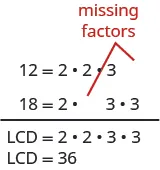The LCD, $36,36,$ has $22$ factors of $22$ and $22$ factors of $3.3.$

Twelve has two factors of $2,2,$ but only one of $33$—so it is ‘missing‘ one $3.3.$ We multiplied the numerator and denominator of $712712$ by $33$ to get an equivalent fraction with denominator $36.36.$

Eighteen is missing one factor of $22$—so you multiply the numerator and denominator $518518$ by $22$ to get an equivalent fraction with denominator $36.36.$ We will apply this method as we subtract the fractions in the next example.

### Example 4.70

Subtract: $715−1924.715−1924.$

### Try It 4.139

Subtract: $1324−1732.1324−1732.$

### Try It 4.140

Subtract: $2132−928.2132−928.$

### Example 4.71

Add: $−1130+2342.−1130+2342.$

### Try It 4.141

Add: $−1342+1735.−1342+1735.$

### Try It 4.142

Add: $−1924+1732.−1924+1732.$

In the next example, one of the fractions has a variable in its numerator. We follow the same steps as when both numerators are numbers.

### Example 4.72

Add: $35+x8.35+x8.$

### Try It 4.143

Add: $y6+79.y6+79.$

### Try It 4.144

Add: $x6+715.x6+715.$

### Identify and Use Fraction Operations

By now in this chapter, you have practiced multiplying, dividing, adding, and subtracting fractions. The following table summarizes these four fraction operations. Remember: You need a common denominator to add or subtract fractions, but not to multiply or divide fractions

### Summary of Fraction Operations

Fraction multiplication: Multiply the numerators and multiply the denominators.

$ab·cd=acbdab·cd=acbd$

Fraction division: Multiply the first fraction by the reciprocal of the second.

$ab÷cd=ab·dcab÷cd=ab·dc$

Fraction addition: Add the numerators and place the sum over the common denominator. If the fractions have different denominators, first convert them to equivalent forms with the LCD.

$ac+bc=a+bcac+bc=a+bc$

Fraction subtraction: Subtract the numerators and place the difference over the common denominator. If the fractions have different denominators, first convert them to equivalent forms with the LCD.

$ac−bc=a−bcac−bc=a−bc$

### Example 4.73

Simplify:

1. $−14+16−14+16$
2. $−14÷16−14÷16$

### Try It 4.145

Simplify each expression:

1. $−34−16−34−16$
2. $−34·16−34·16$

### Try It 4.146

Simplify each expression:

1. $56÷(−14)56÷(−14)$
2. $56−(−14)56−(−14)$

### Example 4.74

Simplify:

1. $5x6−3105x6−310$
2. $5x6·3105x6·310$

### Try It 4.147

Simplify:

1. $(27a−32)36(27a−32)36$
2. $2a32a3$

### Try It 4.148

Simplify:

1. $(24k+25)30(24k+25)30$
2. $24k524k5$

### Use the Order of Operations to Simplify Complex Fractions

In Multiply and Divide Mixed Numbers and Complex Fractions, we saw that a complex fraction is a fraction in which the numerator or denominator contains a fraction. We simplified complex fractions by rewriting them as division problems. For example,

$3458=34÷583458=34÷58$

Now we will look at complex fractions in which the numerator or denominator can be simplified. To follow the order of operations, we simplify the numerator and denominator separately first. Then we divide the numerator by the denominator.

### How To

#### Simplify complex fractions.

1. Step 1. Simplify the numerator.
2. Step 2. Simplify the denominator.
3. Step 3. Divide the numerator by the denominator.
4. Step 4. Simplify if possible.

### Example 4.75

Simplify: $(12)24+32.(12)24+32.$

### Try It 4.149

Simplify: $(13)223+2(13)223+2$.

### Try It 4.150

Simplify: $1+42(14)21+42(14)2$.

### Example 4.76

Simplify: $12+2334−16.12+2334−16.$

### Try It 4.151

Simplify: $13+1234−1313+1234−13$.

### Try It 4.152

Simplify: $23−1214+1323−1214+13$.

### Evaluate Variable Expressions with Fractions

We have evaluated expressions before, but now we can also evaluate expressions with fractions. Remember, to evaluate an expression, we substitute the value of the variable into the expression and then simplify.

### Example 4.77

Evaluate $x+13x+13$ when

1. $x=−13x=−13$
2. $x=−34.x=−34.$

### Try It 4.153

Evaluate: $x+34x+34$ when

1. $x=−74x=−74$
2. $x=−54x=−54$

### Try It 4.154

Evaluate: $y+12y+12$ when

1. $y=23y=23$
2. $y=−34y=−34$

### Example 4.78

Evaluate $y−56y−56$ when $y=−23.y=−23.$

### Try It 4.155

Evaluate: $y−12y−12$ when $y=−14.y=−14.$

### Try It 4.156

Evaluate: $x−38x−38$ when $x=−52.x=−52.$

### Example 4.79

Evaluate $2x2y2x2y$ when $x=14x=14$ and $y=−23.y=−23.$

### Try It 4.157

Evaluate. $3ab23ab2$ when $a=−23a=−23$ and $b=−12.b=−12.$

### Try It 4.158

Evaluate. $4c3d4c3d$ when $c=−12c=−12$ and $d=−43.d=−43.$

### Example 4.80

Evaluate $p+qrp+qr$ when $p=−4,q=−2,p=−4,q=−2,$ and $r=8.r=8.$

### Try It 4.159

Evaluate: $a+bca+bc$ when $a=−8,b=−7,a=−8,b=−7,$ and $c=6.c=6.$

### Try It 4.160

Evaluate: $x+yzx+yz$ when $x=9,y=−18,x=9,y=−18,$ and $z=−6.z=−6.$

### Section 4.5 Exercises

#### Practice Makes Perfect

Find the Least Common Denominator (LCD)

In the following exercises, find the least common denominator (LCD) for each set of fractions.

316.

$2323$ and $3434$

317.

$3434$ and $2525$

318.

$712712$ and $5858$

319.

$916916$ and $712712$

320.

$13301330$ and $25422542$

321.

$23302330$ and $548548$

322.

$21352135$ and $39563956$

323.

$18351835$ and $33493349$

324.

$23,16,23,16,$ and $3434$

325.

$23,14,23,14,$ and $3535$

Convert Fractions to Equivalent Fractions with the LCD

In the following exercises, convert to equivalent fractions using the LCD.

326.

$1313$ and $14,14,$ LCD $=12=12$

327.

$1414$ and $15,15,$ LCD $=20=20$

328.

$512512$ and $78,78,$ LCD $=24=24$

329.

$712712$ and $58,58,$ LCD $=24=24$

330.

$13161316$ and $-1112,-1112,$ LCD $=48=48$

331.

$11161116$ and $-512,-512,$ LCD $=48=48$

332.

$13,56,13,56,$ and $34,34,$ LCD $=12=12$

333.

$13,34,13,34,$ and $35,35,$ LCD $=60=60$

Add and Subtract Fractions with Different Denominators

In the following exercises, add or subtract. Write the result in simplified form.

334.

$1 3 + 1 5 1 3 + 1 5$

335.

$1 4 + 1 5 1 4 + 1 5$

336.

$1 2 + 1 7 1 2 + 1 7$

337.

$1 3 + 1 8 1 3 + 1 8$

338.

$1 3 − ( − 1 9 ) 1 3 − ( − 1 9 )$

339.

$1 4 − ( − 1 8 ) 1 4 − ( − 1 8 )$

340.

$1 5 − ( − 1 10 ) 1 5 − ( − 1 10 )$

341.

$1 2 − ( − 1 6 ) 1 2 − ( − 1 6 )$

342.

$2 3 + 3 4 2 3 + 3 4$

343.

$3 4 + 2 5 3 4 + 2 5$

344.

$7 12 + 5 8 7 12 + 5 8$

345.

$5 12 + 3 8 5 12 + 3 8$

346.

$7 12 − 9 16 7 12 − 9 16$

347.

$7 16 − 5 12 7 16 − 5 12$

348.

$11 12 − 3 8 11 12 − 3 8$

349.

$5 8 − 7 12 5 8 − 7 12$

350.

$2 3 − 3 8 2 3 − 3 8$

351.

$5 6 − 3 4 5 6 − 3 4$

352.

$− 11 30 + 27 40 − 11 30 + 27 40$

353.

$− 9 20 + 17 30 − 9 20 + 17 30$

354.

$− 13 30 + 25 42 − 13 30 + 25 42$

355.

$− 23 30 + 5 48 − 23 30 + 5 48$

356.

$− 39 56 − 22 35 − 39 56 − 22 35$

357.

$− 33 49 − 18 35 − 33 49 − 18 35$

358.

$− 2 3 − ( − 3 4 ) − 2 3 − ( − 3 4 )$

359.

$− 3 4 − ( − 4 5 ) − 3 4 − ( − 4 5 )$

360.

$− 9 16 − ( − 4 5 ) − 9 16 − ( − 4 5 )$

361.

$− 7 20 − ( − 5 8 ) − 7 20 − ( − 5 8 )$

362.

$1 + 7 8 1 + 7 8$

363.

$1 + 5 6 1 + 5 6$

364.

$1 − 5 9 1 − 5 9$

365.

$1 − 3 10 1 − 3 10$

366.

$x 3 + 1 4 x 3 + 1 4$

367.

$y 2 + 2 3 y 2 + 2 3$

368.

$y 4 − 3 5 y 4 − 3 5$

369.

$x 5 − 1 4 x 5 − 1 4$

Identify and Use Fraction Operations

In the following exercises, perform the indicated operations. Write your answers in simplified form.

370.
1. $34+1634+16$
2. $34÷1634÷16$
371.
1. $23+1623+16$
2. $23÷1623÷16$
372.
1. $-25−18-25−18$
2. $-25·18-25·18$
373.
1. $-45−18-45−18$
2. $-45·18-45·18$
374.
1. $5n6÷8155n6÷815$
2. $5n6−8155n6−815$
375.
1. $3a8÷7123a8÷712$
2. $3a8−7123a8−712$
376.
1. $910·(−11d12)910·(−11d12)$
2. $910+(−11d12)910+(−11d12)$
377.
1. $415·(−5q9)415·(−5q9)$
2. $415+(−5q9)415+(−5q9)$
378.

$− 3 8 ÷ ( − 3 10 ) − 3 8 ÷ ( − 3 10 )$

379.

$− 5 12 ÷ ( − 5 9 ) − 5 12 ÷ ( − 5 9 )$

380.

$− 3 8 + 5 12 − 3 8 + 5 12$

381.

$− 1 8 + 7 12 − 1 8 + 7 12$

382.

$5 6 − 1 9 5 6 − 1 9$

383.

$5 9 − 1 6 5 9 − 1 6$

384.

$3 8 · ( − 10 21 ) 3 8 · ( − 10 21 )$

385.

$7 12 · ( − 8 35 ) 7 12 · ( − 8 35 )$

386.

$− 7 15 − y 4 − 7 15 − y 4$

387.

$− 3 8 − x 11 − 3 8 − x 11$

388.

$11 12 a · 9 a 16 11 12 a · 9 a 16$

389.

$10 y 13 · 8 15 y 10 y 13 · 8 15 y$

Use the Order of Operations to Simplify Complex Fractions

In the following exercises, simplify.

390.

$( 1 5 ) 2 2 + 3 2 ( 1 5 ) 2 2 + 3 2$

391.

$( 1 3 ) 2 5 + 2 2 ( 1 3 ) 2 5 + 2 2$

392.

$2 3 + 4 2 ( 2 3 ) 2 2 3 + 4 2 ( 2 3 ) 2$

393.

$3 3 − 3 2 ( 3 4 ) 2 3 3 − 3 2 ( 3 4 ) 2$

394.

$( 3 5 ) 2 ( 3 7 ) 2 ( 3 5 ) 2 ( 3 7 ) 2$

395.

$( 3 4 ) 2 ( 5 8 ) 2 ( 3 4 ) 2 ( 5 8 ) 2$

396.

$2 1 3 + 1 5 2 1 3 + 1 5$

397.

$5 1 4 + 1 3 5 1 4 + 1 3$

398.

$2 3 + 1 2 3 4 − 2 3 2 3 + 1 2 3 4 − 2 3$

399.

$3 4 + 1 2 5 6 − 2 3 3 4 + 1 2 5 6 − 2 3$

400.

$7 8 − 2 3 1 2 + 3 8 7 8 − 2 3 1 2 + 3 8$

401.

$3 4 − 3 5 1 4 + 2 5 3 4 − 3 5 1 4 + 2 5$

Mixed Practice

In the following exercises, simplify.

402.

$1 2 + 2 3 · 5 12 1 2 + 2 3 · 5 12$

403.

$1 3 + 2 5 · 3 4 1 3 + 2 5 · 3 4$

404.

$1 − 3 5 ÷ 1 10 1 − 3 5 ÷ 1 10$

405.

$1 − 5 6 ÷ 1 12 1 − 5 6 ÷ 1 12$

406.

$2 3 + 1 6 + 3 4 2 3 + 1 6 + 3 4$

407.

$2 3 + 1 4 + 3 5 2 3 + 1 4 + 3 5$

408.

$3 8 − 1 6 + 3 4 3 8 − 1 6 + 3 4$

409.

$2 5 + 5 8 − 3 4 2 5 + 5 8 − 3 4$

410.

$12 ( 9 20 − 4 15 ) 12 ( 9 20 − 4 15 )$

411.

$8 ( 15 16 − 5 6 ) 8 ( 15 16 − 5 6 )$

412.

$5 8 + 1 6 19 24 5 8 + 1 6 19 24$

413.

$1 6 + 3 10 14 30 1 6 + 3 10 14 30$

414.

$( 5 9 + 1 6 ) ÷ ( 2 3 − 1 2 ) ( 5 9 + 1 6 ) ÷ ( 2 3 − 1 2 )$

415.

$( 3 4 + 1 6 ) ÷ ( 5 8 − 1 3 ) ( 3 4 + 1 6 ) ÷ ( 5 8 − 1 3 )$

In the following exercises, evaluate the given expression. Express your answers in simplified form, using improper fractions if necessary.

416.

$x+12x+12$ when

1. $x=−18x=−18$
2. $x=−12x=−12$
417.

$x+23x+23$ when

1. $x=−16x=−16$
2. $x=−53x=−53$
418.

$x+(−56)x+(−56)$ when

1. $x=13x=13$
2. $x=−16x=−16$
419.

$x+(−1112)x+(−1112)$ when

1. $x=1112x=1112$
2. $x=34x=34$
420.

$x−25x−25$ when

1. $x=35x=35$
2. $x=−35x=−35$
421.

$x−13x−13$ when

1. $x=23x=23$
2. $x=−23x=−23$
422.

$710−w710−w$ when

1. $w=12w=12$
2. $w=−12w=−12$
423.

$512−w512−w$ when

1. $w=14w=14$
2. $w=−14w=−14$
424.

$4p2q4p2q$ when $p=−12p=−12$ and $q=59q=59$

425.

$5m2n5m2n$ when $m=−25m=−25$ and $n=13n=13$

426.

$2x2y32x2y3$ when $x=−23 x=−23$ and $y=−12 y=−12$

427.

$8u2v38u2v3$ when $u=−34u=−34$ and $v=−12v=−12$

428.

$u+vwu+vw$ when $u=−4,v=−8,w=2u=−4,v=−8,w=2$

429.

$m+npm+np$ when $m=−6,n=−2,p=4m=−6,n=−2,p=4$

430.

$a+ba−ba+ba−b$ when $a=−3,b=8a=−3,b=8$

431.

$r−sr+sr−sr+s$ when $r=10,s=−5r=10,s=−5$

#### Everyday Math

432.

Decorating Laronda is making covers for the throw pillows on her sofa. For each pillow cover, she needs $316316$ yard of print fabric and $3838$ yard of solid fabric. What is the total amount of fabric Laronda needs for each pillow cover?

433.

Baking Vanessa is baking chocolate chip cookies and oatmeal cookies. She needs $114114$ cups of sugar for the chocolate chip cookies, and $118118$ cups for the oatmeal cookies How much sugar does she need altogether?

#### Writing Exercises

434.

Explain why it is necessary to have a common denominator to add or subtract fractions.

435.

Explain how to find the LCD of two fractions.

#### Self Check

After completing the exercises, use this checklist to evaluate your mastery of the objectives of this section.

After looking at the checklist, do you think you are well prepared for the next section? Why or why not?

Order a print copy

As an Amazon Associate we earn from qualifying purchases.